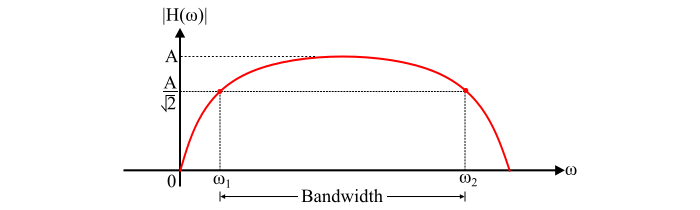# Signals and Systems – System Bandwidth Vs. Signal Bandwidth

Signals and SystemsElectronics & ElectricalDigital Electronics

## Signal Bandwidth

The spectral components of a signal extends from (−∞) to ∞ and any practical signal has finite energy. Consequently, the spectral components approach zero when the frequency 𝜔 tends to ∞. Therefore, those spectral components can be neglected which have negligible energy and hence only a band of frequency components is selected which have most of the signal energy. This band of frequency components which contains most of the signal energy is called the signal bandwidth.

Normally, this band has the frequency components that contains around 95% of the total energy depending upon the precision.

## System Bandwidth

A system which has infinite bandwidth can transmit the signals without any distortion. But, due to physical limitations, no system can be constructed that has infinite bandwidth. However, in practice, a satisfactory distortion-less transmission can be achieved by a system with finite bandwidth, but the bandwidth should be fairly large, i.e., if the magnitude |𝐻(𝜔)| is constant over the band, then the distortion less transmission of the signals can be achieved from the system.

The bandwidth of the system specifies the constancy of the magnitude of the transfer function |𝐻(𝜔)|. Therefore, the bandwidth of a system can be defined as follows −

The range of frequencies over which the magnitude |𝐻(𝜔)| remains within (1⁄√2) or within 3 dB of its maximum value or the value at mid-band is called the bandwidth of the system. The plot of the bandwidth of a system is shown in the figure.From the figure,

Bandwidth = 𝜔2 − 𝜔1

Where,

• 𝜔1 is called the lower cut-off frequency or lower half-power frequency or lower 3 dB frequency.

• 𝜔2 is called the upper cut-off frequency or upper half-power frequency or upper 3 dB frequency.

Note – When the bandwidth of a system is at least equal to the bandwidth of the signal, then the band limited signals can be transmitted without distortion through the system.